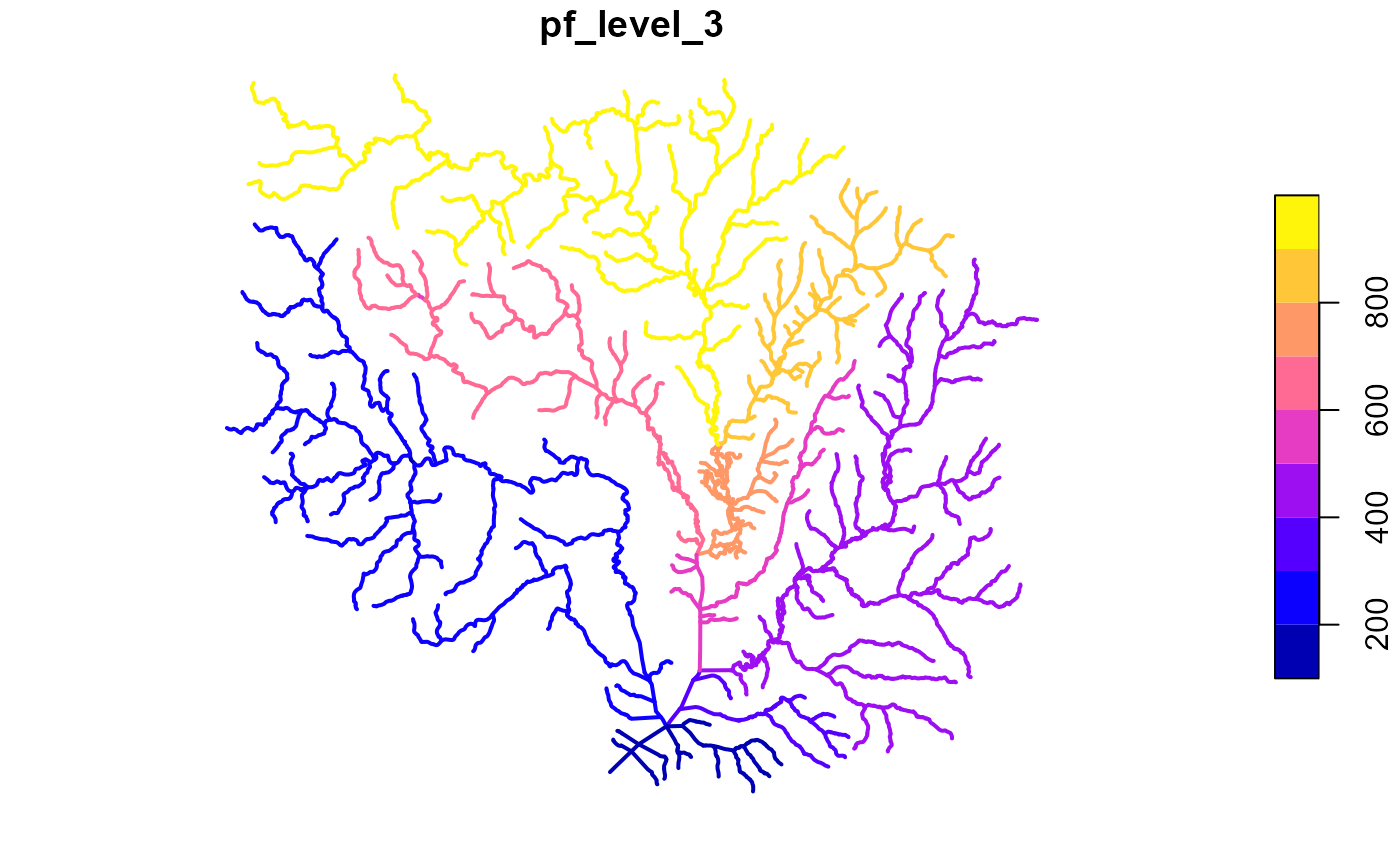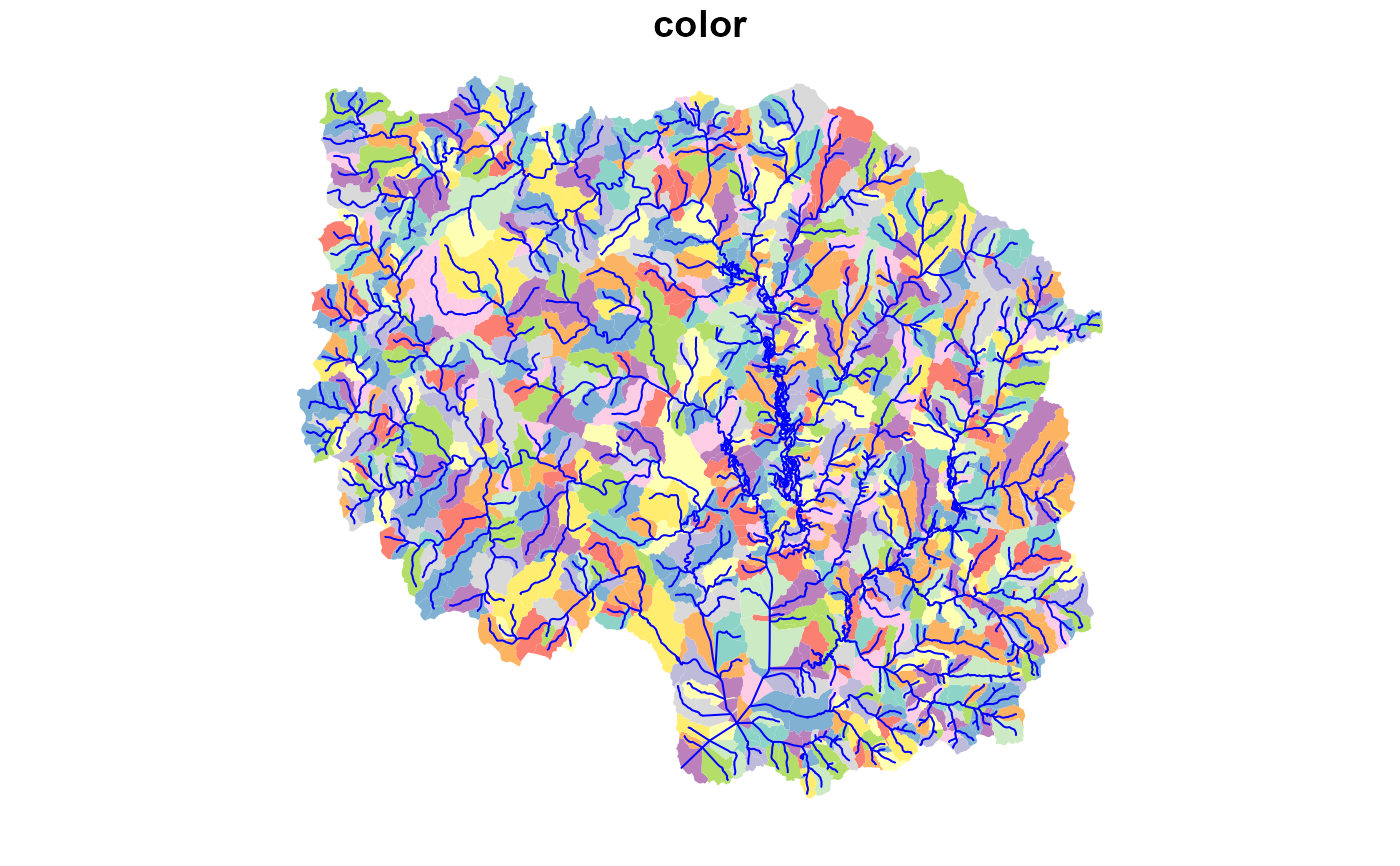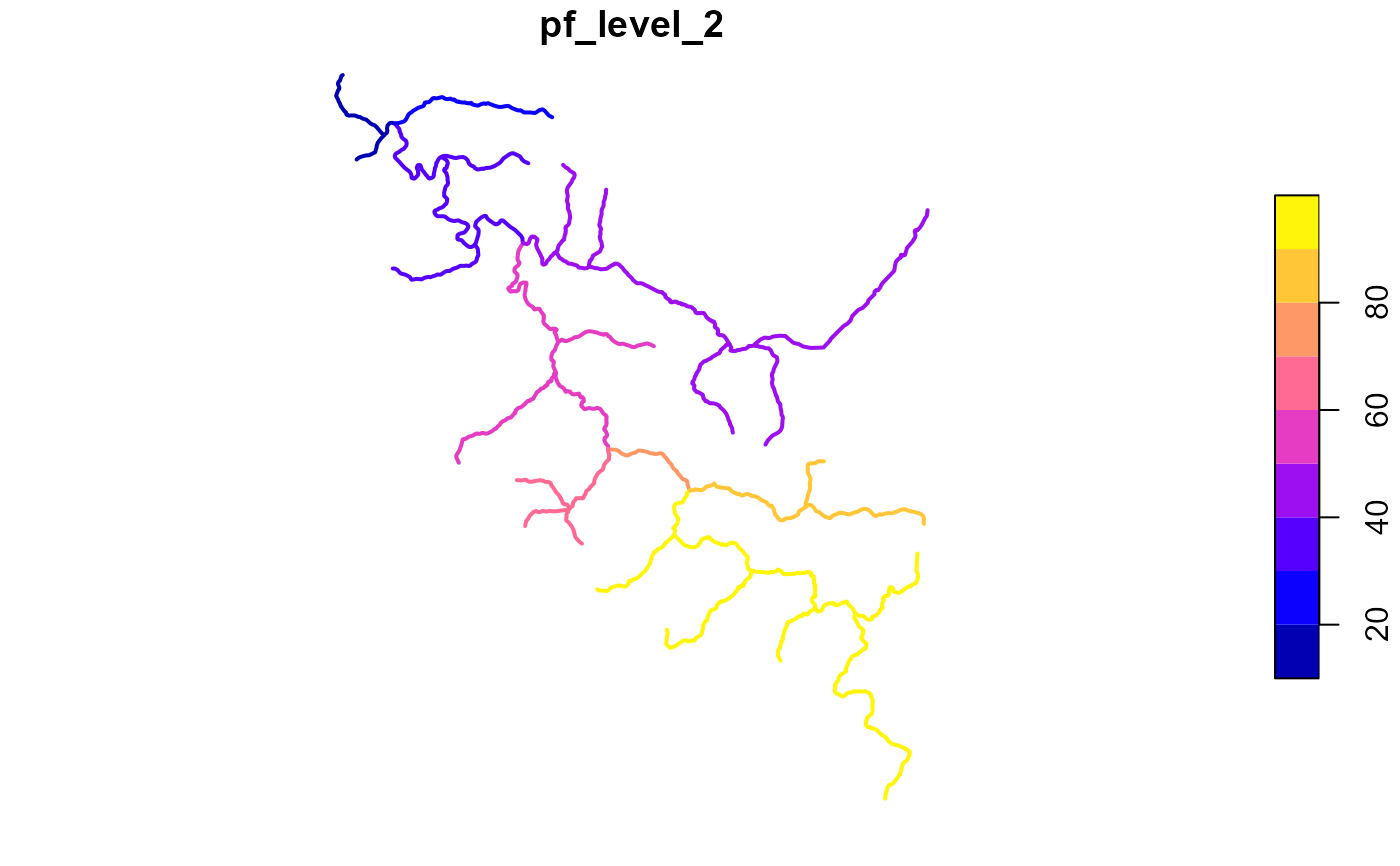Determines Pfafstetter codes for a dendritic network with total drainage area, levelpath, and topo_sort attributes.

get_pfaf(x, max_level = 2, status = FALSE)

## Arguments

x

sf data.frame with ID, toID, totda, outletID, topo_sort, and levelpath attributes.

max_level

integer number of pfaf levels to attempt to calculate. If the network doesn't have resolution to support the desired level, unexpected behavior may occur.

status

boolean print status or not

## Value

data.frame with ID and pfaf columns.

## Examples

# \donttest{
library(dplyr)
source(system.file("extdata/nhdplushr_data.R", package = "nhdplusTools"))
hr_flowline <- align_nhdplus_names(hr_data$NHDFlowline) fl <- select(hr_flowline, COMID, AreaSqKM) %>% right_join(prepare_nhdplus(hr_flowline, 0, 0, purge_non_dendritic = FALSE, warn = FALSE), by = "COMID") %>% sf::st_sf() %>% select(ID = COMID, toID = toCOMID, area = AreaSqKM) fl$nameID = ""
fl$totda <- calculate_total_drainage_area(sf::st_set_geometry(fl, NULL)) fl <- left_join(fl, get_levelpaths(rename(sf::st_set_geometry(fl, NULL), weight = totda)), by = "ID") #> Warning: get_levelpaths is deprecated in favor of add_levelpaths in the hydroloom package. pfaf <- get_pfaf(fl, max_level = 3) #> Warning: get_pfaf is deprecated, please use hydroloom fl <- left_join(fl, pfaf, by = "ID") plot(fl["pf_level_3"], lwd = 2)pfaf <- get_pfaf(fl, max_level = 4) #> Warning: get_pfaf is deprecated, please use hydroloom hr_catchment <- left_join(hr_data$NHDPlusCatchment, pfaf, by = c("FEATUREID" = "ID"))

colors <- data.frame(pf_level_4 = unique(hr_catchment$pf_level_4), color = sample(terrain.colors(length(unique(hr_catchment$pf_level_4)))),
stringsAsFactors = FALSE)
hr_catchment <- left_join(hr_catchment, colors, by = "pf_level_4")
plot(hr_catchment["color"], border = NA, reset = FALSE)
plot(sf::st_geometry(hr_flowline), col = "blue", add = TRUE)source(system.file("extdata", "walker_data.R", package = "nhdplusTools"))

fl <- select(walker_flowline, COMID, AreaSqKM) %>%
right_join(prepare_nhdplus(walker_flowline, 0, 0,
purge_non_dendritic = FALSE, warn = FALSE),
by = "COMID") %>%
sf::st_sf() %>%
select(ID = COMID, toID = toCOMID, area = AreaSqKM)

fl$nameID = "" fl$totda <- calculate_total_drainage_area(sf::st_set_geometry(fl, NULL))
fl <- left_join(fl, get_levelpaths(rename(sf::st_set_geometry(fl, NULL),
weight = totda)), by = "ID")
#> Warning: get_levelpaths is deprecated in favor of add_levelpaths in the hydroloom package.

pfaf <- get_pfaf(fl, max_level = 2)
#> Warning: get_pfaf is deprecated, please use hydroloom

fl <- left_join(fl, pfaf, by = "ID")

plot(fl["pf_level_2"], lwd = 2)# }